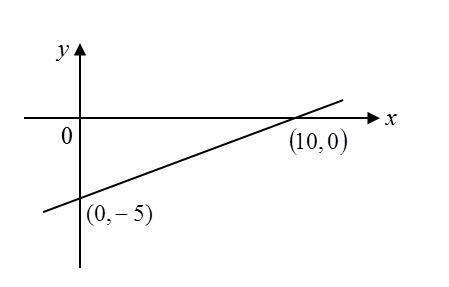\

# 7.4.1 Equations of Straight Lines (Part 1)

2.
Gradient of the line with knowing x–intercept and y–intercept
is:

3.
The gradient of the straight line joining P and Q is equal to the tangent of angle θ, where θ is the angle made by the straight line PQ and the positive direction of the x-axis.(B) Collinear points
The gradient of a straight line is always constant i.e.the gradient of AB is equal to the gradient of BC.Example 1:
The gradient of the line passing through point (k, 1 – k) and point (–3k, –3) is 5.  Find the value of k.

Solution:

Example 2:

Based on the diagram below, find the gradient of the line.Solution: